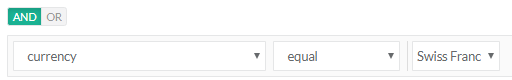This topic contains a set of tables that describe fields, value types, and operators you use when building a plain query.

## TokensValue Description
Fields The available fields may include SKU, item-price, item-quantity or a variety of attributes (e.g. currency on the image above).
Operator The operator compares the value of a field on the left with the value(s) on the right (e.g. equals ‘=’, greater than ‘>’). If the expression evaluates to true, the discount can be applied. (operator is equal on the image above)
Value The value types must match the selected field. The asterisk (*) matches all possible values. (on the image above, the value is Swiss Franc)
Combine Conditions ‘AND’ and ‘OR’ operators are used to combine conditions. (AND on the image above)
Grouping When building more complex queries, conditions may be grouped inside parentheses ‘( )’.

## Fields and value types (Plain Query)

Field Plain Query Value Type Description
Calendar week calender-week Number Week number in a year (1-52)
Day of week day-of-week Number Day of week (1-7)
Grand total grand-total Number (Decimal) The sum of all totals
Subtotal sub-total Number (Decimal) The sum of item prices w/o shipment expenses and discounts
Item price item-price Number (Decimal) The price of one item
Item quantity item-quantity Number The number of items
Month month Number The month of the year (1-12)
SKU sku String Any value depends on how SKUs are stored
Time time hour:minute Time of the day
Total quantity total-quantity Number Total cart quantity
Attribute attribute.* String, number Any value
Customer Group customer-group String Any value, use a customer group name for an exact match

## Operators (Plain Query)

Operator Operator for plain query Value type Description
Contains CONTAINS String, Number Checks if the value is contained in the field
Doesn’t contain DOES NOT CONTAIN String, Number Checks if the value is not contained in the field
Equal = String, Number Checks if the value is equal to the value of the right operand
Not Equal != String, Number Checks if the value is not equal to the value of the right operand
In IS IN List Values need to be semicolon separated
Not In IS NOT IN List Values need to be semicolon separated
Less < Number Checks if the value is less than the value of the right operand
Less or equal <= Number Checks if the value is less than or equal to the value of the right operand
Greater > Number Checks if the value is greater than the value of the right operand
Greater or equal >= Number Checks if the value is greater than or equal to the value of the right operand